#Function Repository Resource:

# RecordsSummary

Summarizes datasets, lists, or associations that can be transformed into full two dimensional arrays

Contributed by: Anton Antonov
 ResourceFunction["RecordsSummary"][data] summarizes the argument data. ResourceFunction["RecordsSummary"][data,cols] summarizes data using the specified column names cols.

## Details and Options

ResourceFunction["RecordsSummary"] works on datasets that 2D tables of atomic objects, full 2D arrays (matrices), lists of atomic objects, and associations the values of which are vectors or full 2D arrays.
Missing values are summarized separately.
The number of the summarized categorical values shown in the summary can be with changed with the option settng "MaxTallies"_Integer.
ResourceFunction["RecordsSummary"] threads for a list of rules or an association with the option setting .
For 2D full arrays by default ResourceFunction["RecordsSummary"] automatically names the columns.
For 2D full arrays, lists and associations, a second argument can be provided specifying the column names.
By default the summarized columns are numbered.
Automatic numbering can be prevented with the option setting "NumberedColumns"False.
If v is a vector (that is, has only one dimension) then ResourceFunction["RecordsSummary"][v] is equivalent to ResourceFunction["RecordsSummary"][List/@v].

## Examples

### Basic Examples (5)

Summarize a vector of numbers:

 In:=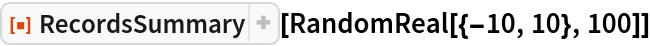Out=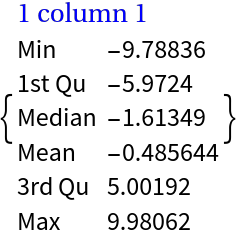Summarize a matrix of strings and specify the column names:

 In:=Out=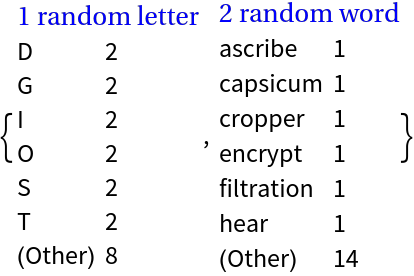Summarize a vector of numbers with missing values:

 In:=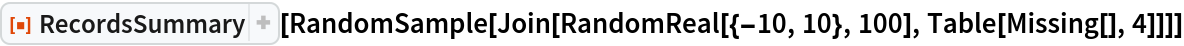Out=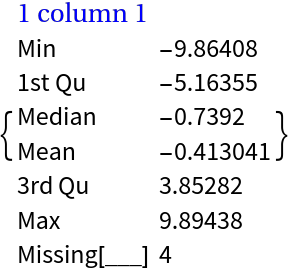Summarize a full 2D array with numerical and categorical columns (numbers, strings, and symbols):

 In:=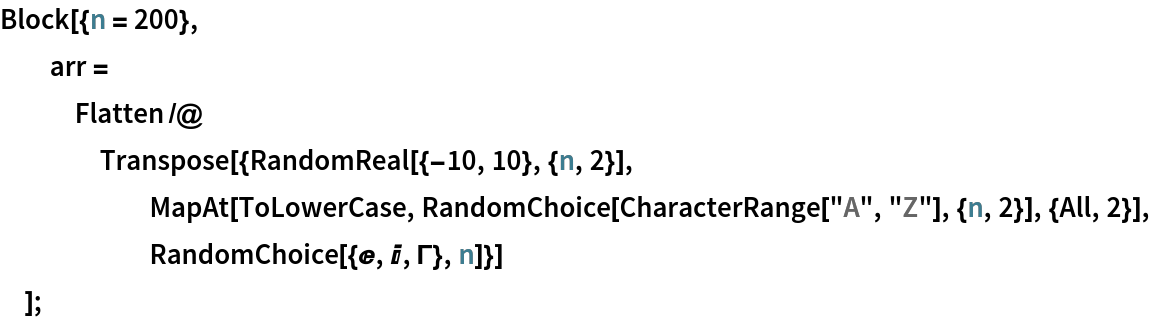In:=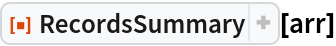Out=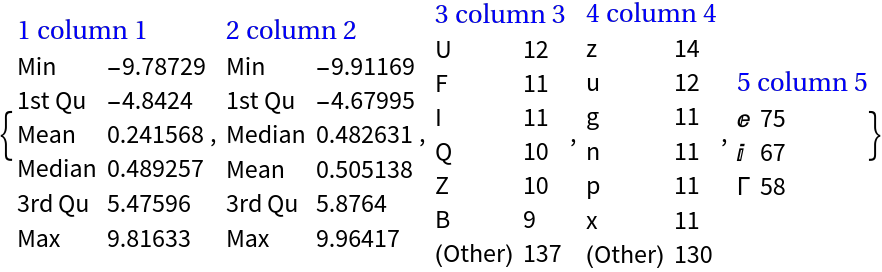Summarize a dataset:

 In:=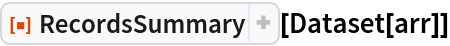Out=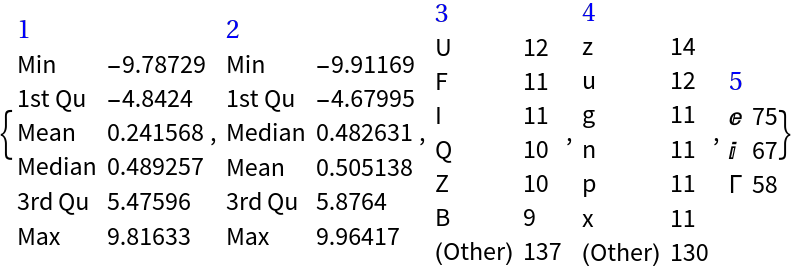Summarize a dataset with column names:

 In:=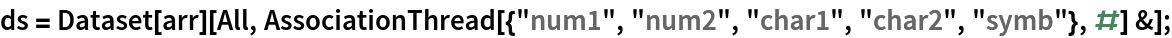In:=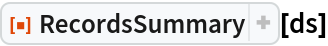Out=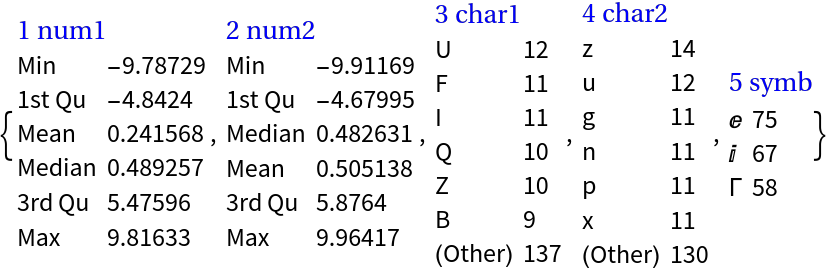Summarize an association of vectors:

 In:=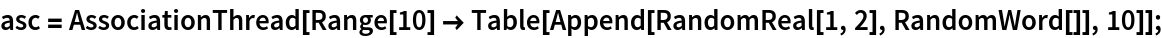In:=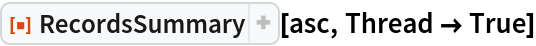Out=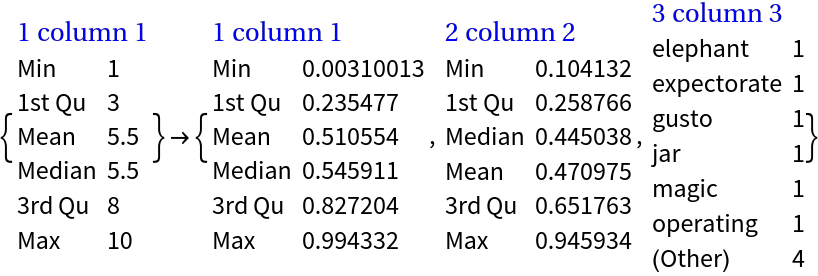### Scope (4)

Define a dataset:

 In:=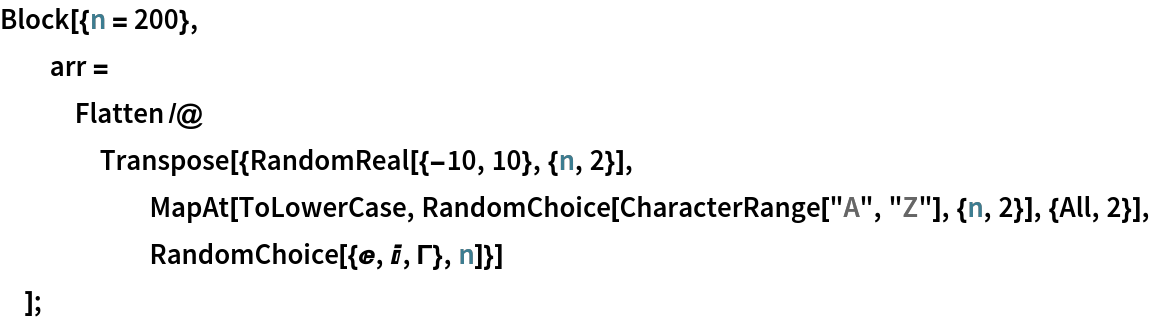In:=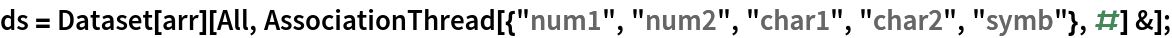A larger number of categorical values can be seen using the option "MaxTallies":

 In:=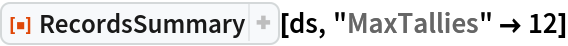Out=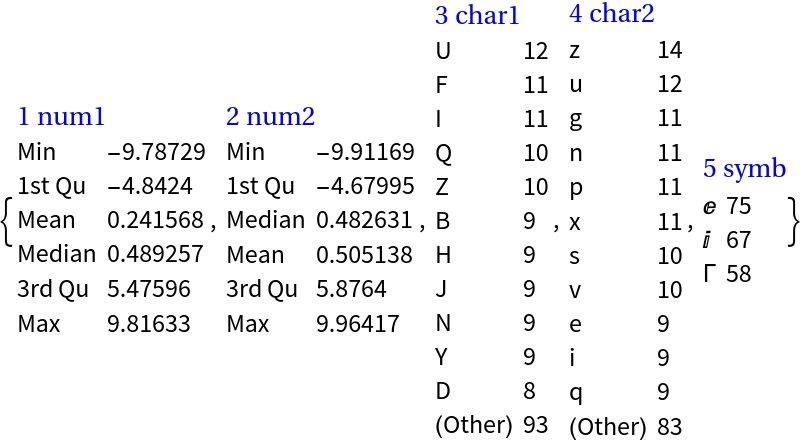The function works with missing values and summarizes them separately of the rest of the values in a column:

 In:=Out=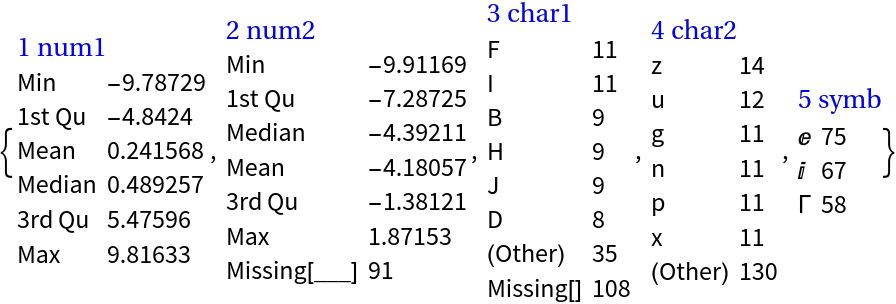Here we make a list of date objects with missing values:

 In:=Here is the summary of the date objects list and with a specified column name:

 In:=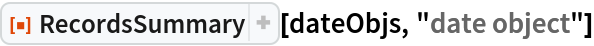Out=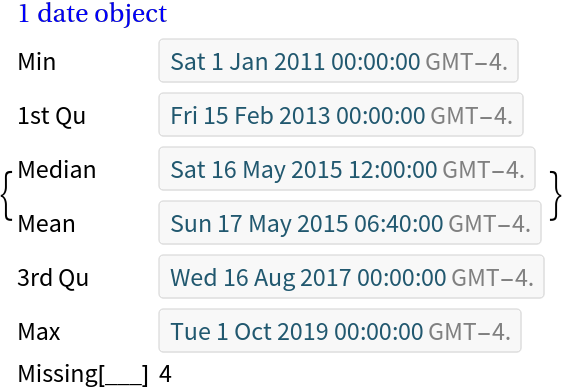Here we make an association of random images:

 In:=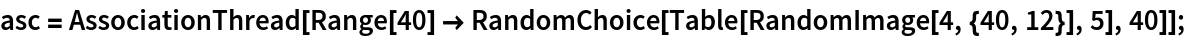This summarizes the list of rules in the association:

 In:=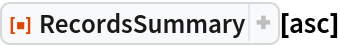Out=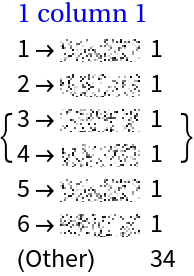We can summarize association’s keys and values separately using the option setting :

 In:=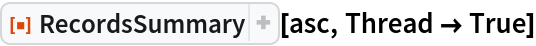Out=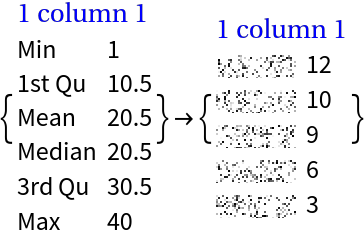A dataset does not have to have named columns:

 In:=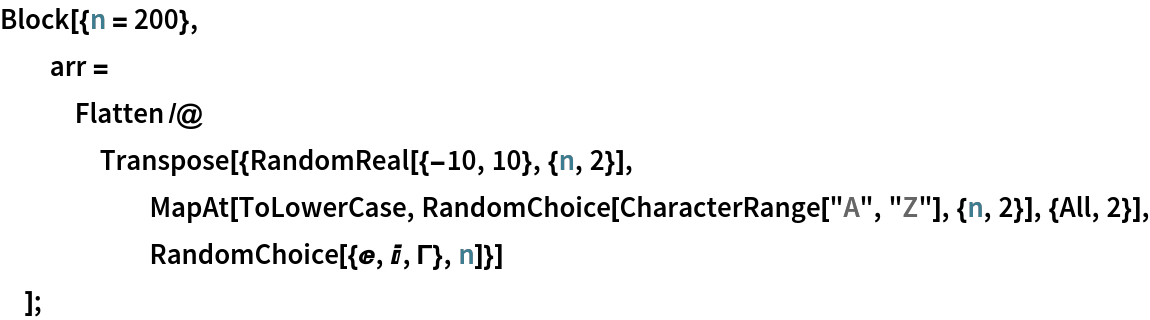In:=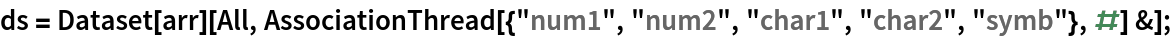In:=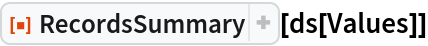Out=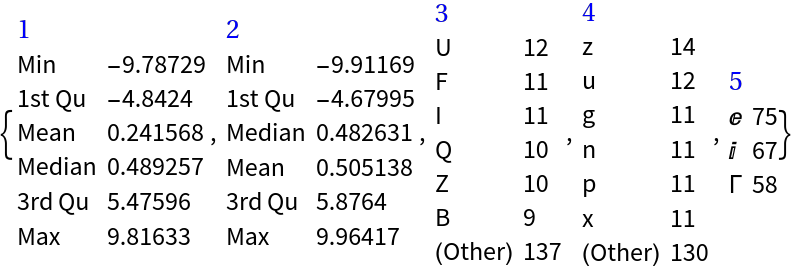### Options (7)

#### MaxTallies (1)

With the option "MaxTallies" we specify how many of summarized items we want to see for each column (variable):

 In:=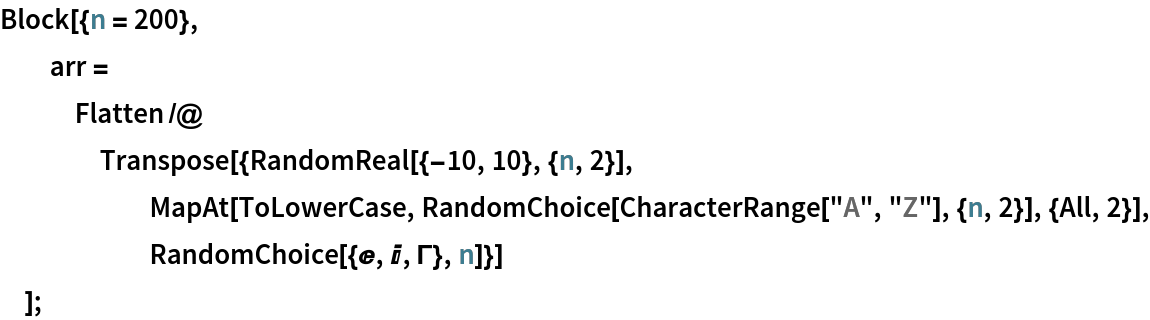In:=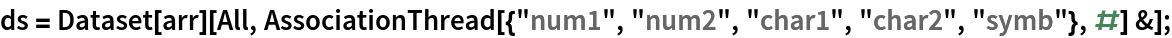In:=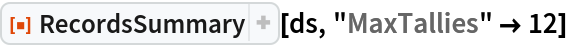Out=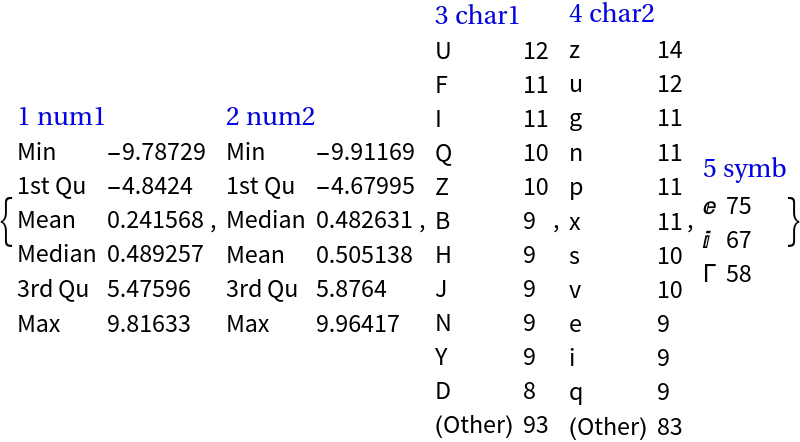In:=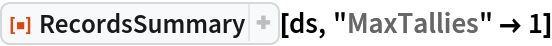Out=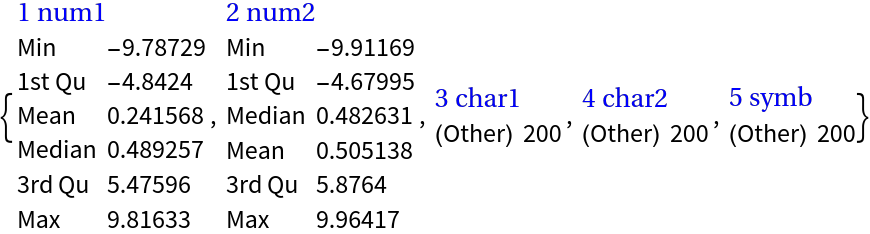#### NumberedColumns (2)

By default the summarized columns (variables) are automatically numbered:

 In:=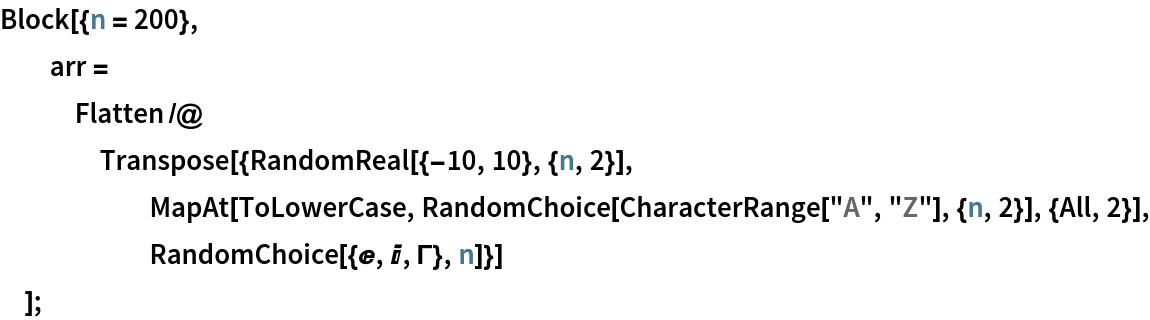In:=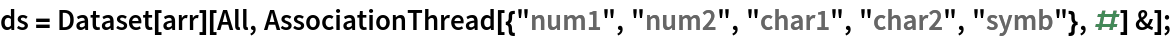In:=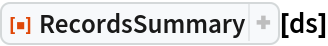Out=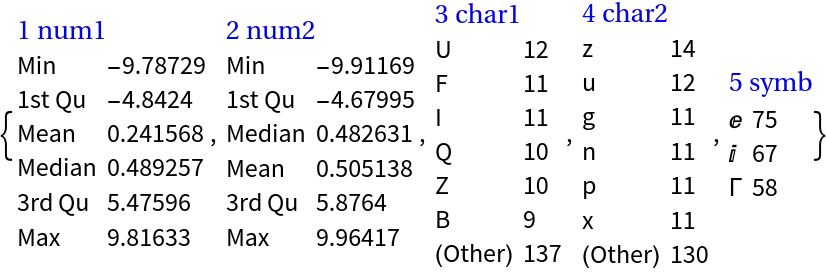With the option "NumberedColumns" the automatic numbering can be prevented:

 In:=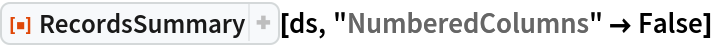Out=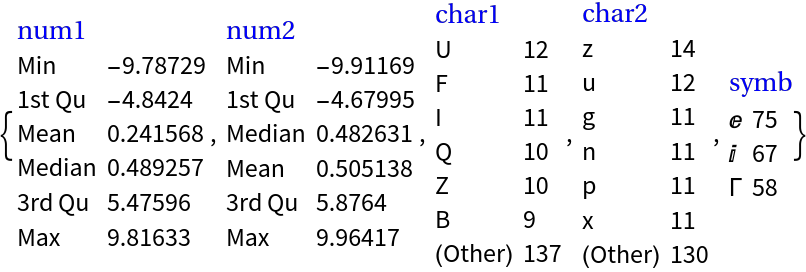The option Thread is used to specify should the summarization be "threaded" if data to be summarized is an association or a list of rules.

Here is an association of 3D points:

 In:=Out=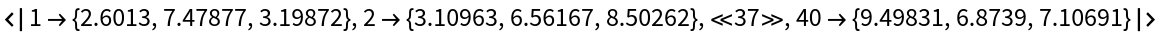In:=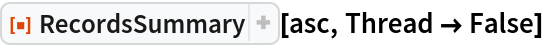Out=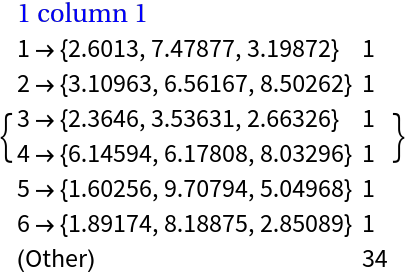In:=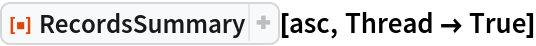Out=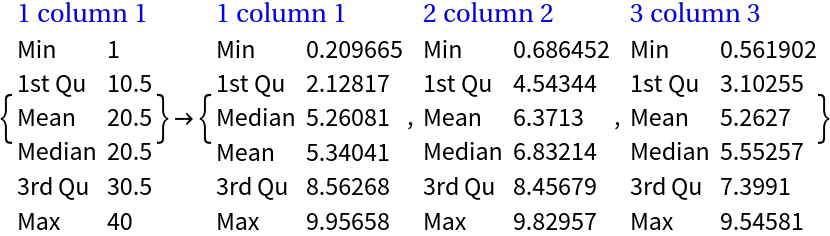Optionally column names can be added:

 In:=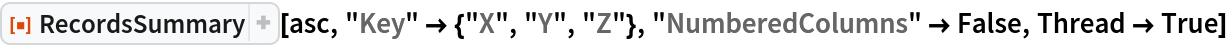Out=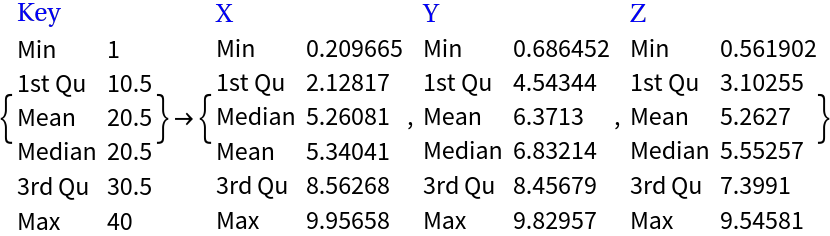### Applications (3)

#### Summarize Classify-ready data (2)

Here we summarize the Titanic data:

 In:=Out=Here we summarize the Mushroom data:

 In:=Out=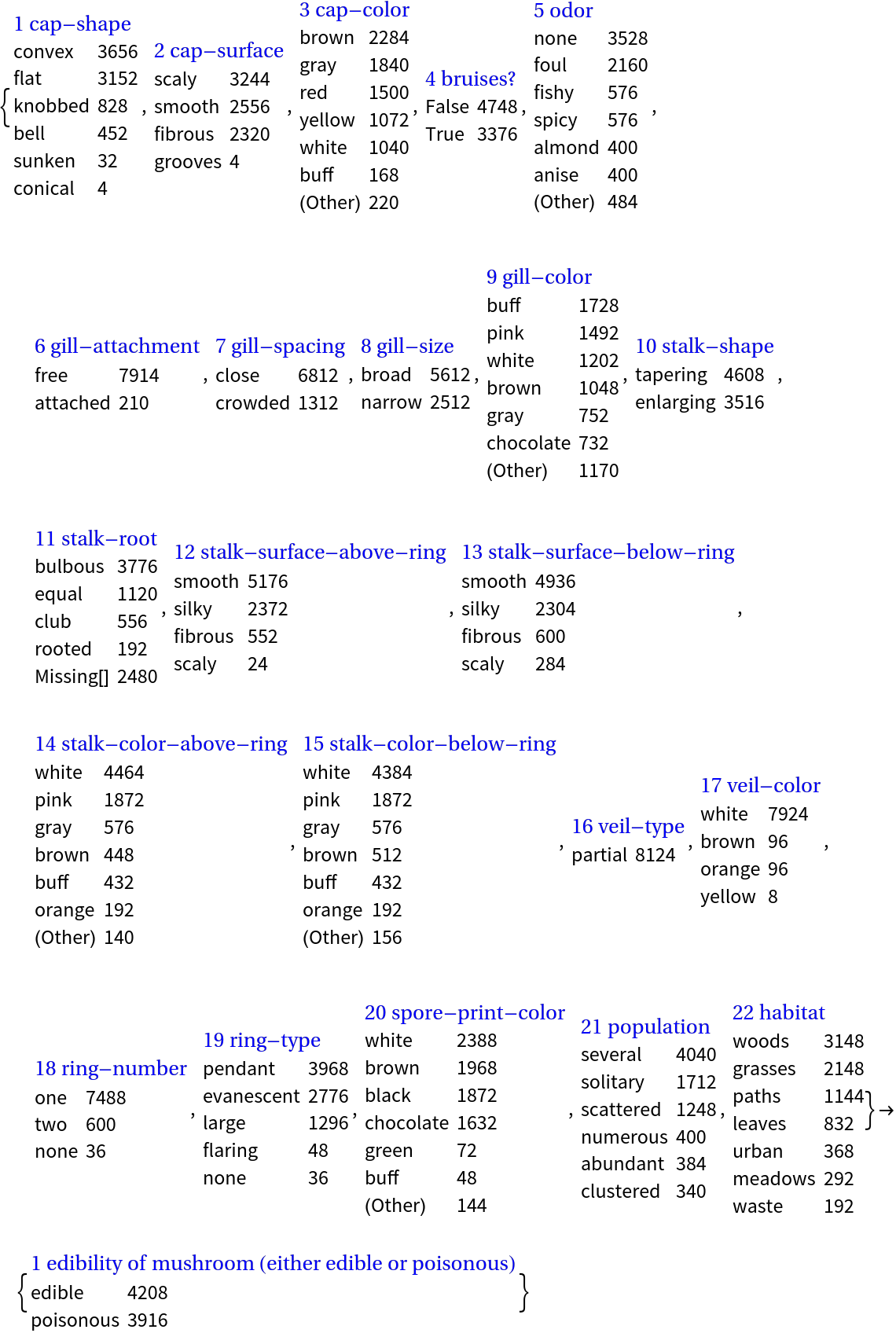#### Summaries browser (1)

If we have a set of datasets we can easily build an interactive interface that allows browsing of dataset summaries:

 In:=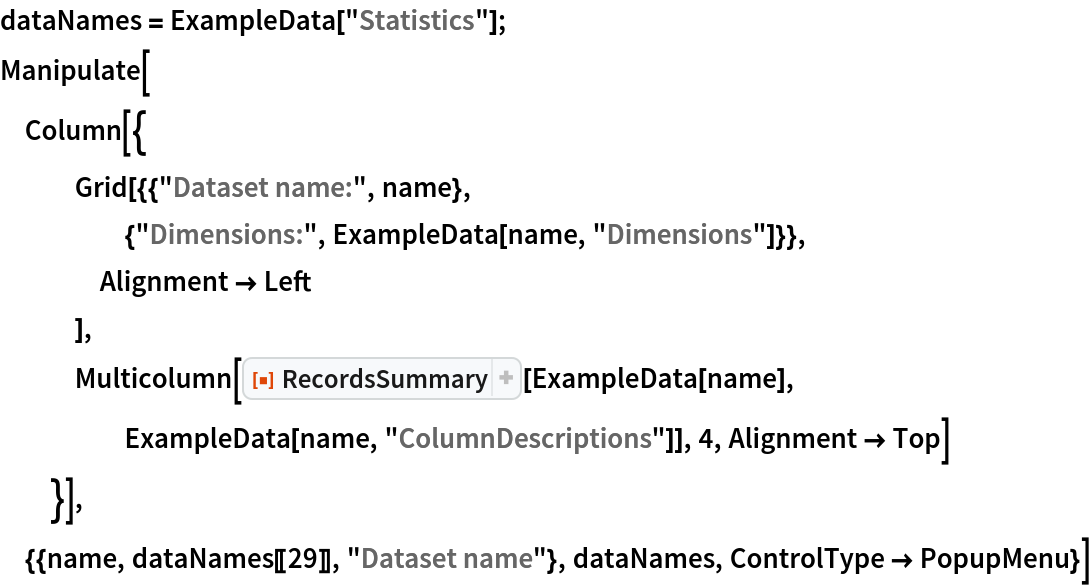Out=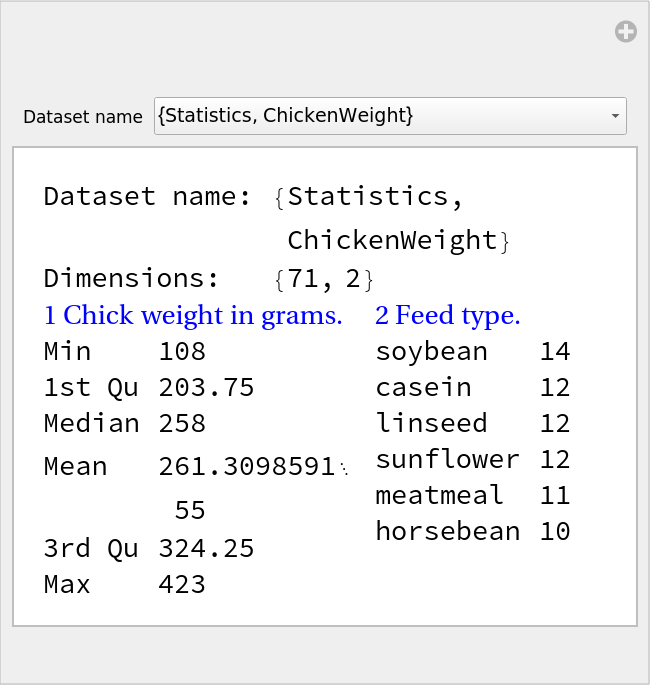### Possible Issues (7)

It is expected that the first argument of RecordsSummary is an object that can be converted to a full array atom objects:

 In:=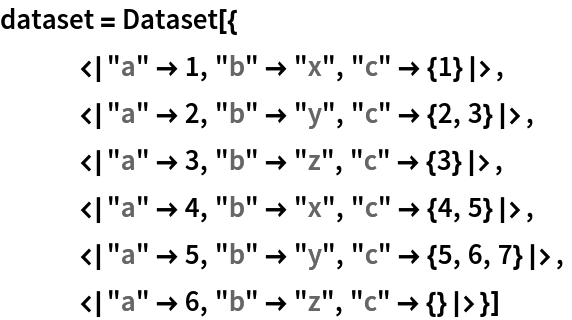Out=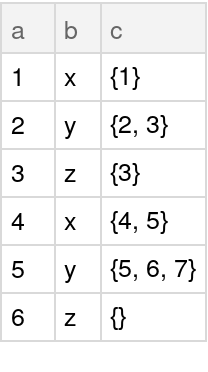This fails because dataset cannot be converted to a full 2D array:

 In:=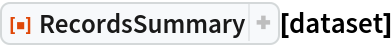Out=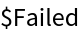A work-around is to use HoldForm for the columns that are not vectors:

 In:=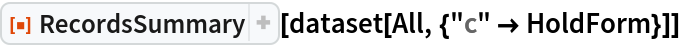Out=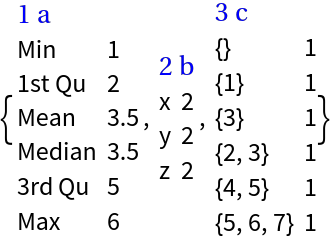If the numerical columns have Quantity values those columns are treated as categorical:

 In:=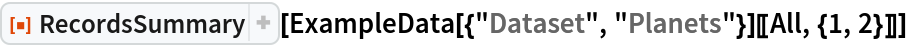Out=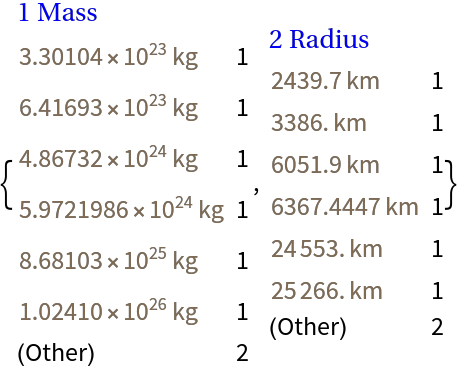A summary of numerical values can be obtained by using QuantityMagnitude:

 In:=Out=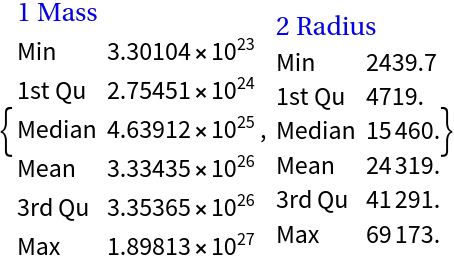For associations the values of which are not full arrays, using the option setting produces a failure:

 In:=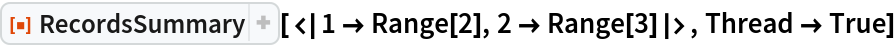Out=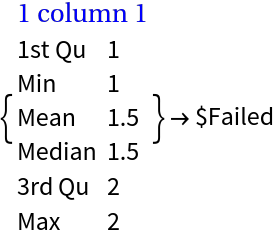This works though:

 In:=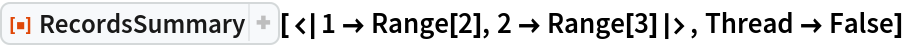Out=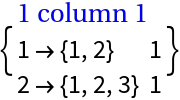### Neat Examples (1)

Summarize subsets of Titanic data that correspond to each passenger class:

 In:=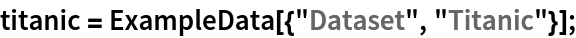In:=Out=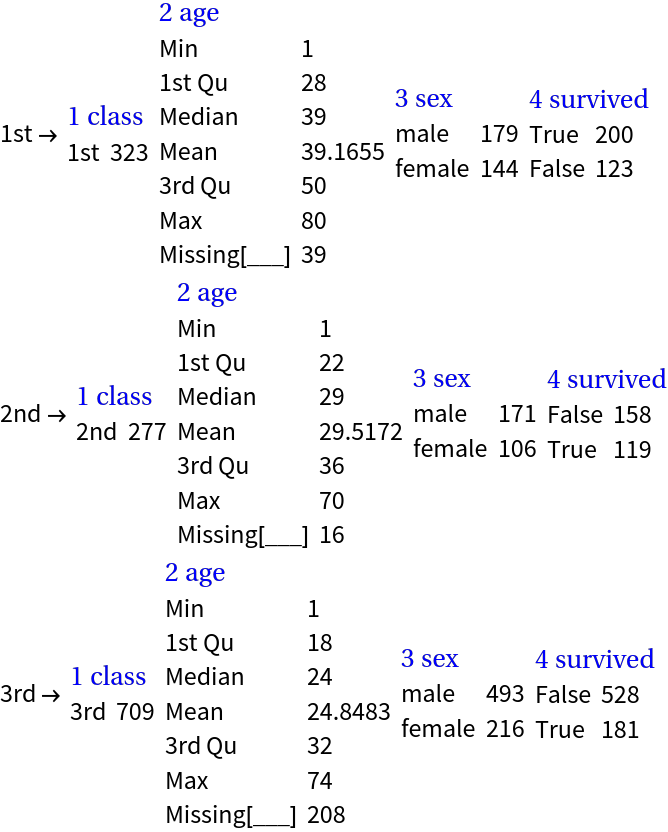Anton Antonov

## Version History

• 1.0.0 – 02 October 2019

## Author Notes

This function, RecordsSummary, corresponds to R’s fundamental function summary.